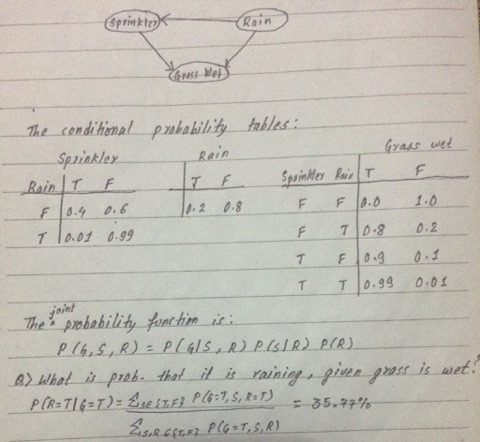### Notes of Artificial Intelligence [CT 653]

#### Knowledge Representation, Inference and Reasoning

Formal Logic

Knowledge representation is the process of expressing the knowledge about the world in a computer tractable form . a formal language represents knowledge into a computer tractable form and the reasoning process manipulate it to deduce non- obvious facts.

Logic

- Logic is a formal language to express knowledge and ways of reasoning that makes statements about the world which may be either truth or false but not both at a time. It is concise unambiguous, context insensitive, expressive and effective for inference
- A logic is defined by:
a) Syntax-> describe possible configurations that constitute sentences.
b) Semantics-> determine the interpretation of sentence about the world.
c) Proof theory ->sets of rules to generate new sentence that are true if old sentences are true.

Propositional and Predicate logic, FOPL, Horn Clauses

Propositional logic

- It is a declarative sentence which can be either true or false but not both.
- It provides a mathematical model to reason about truth or false of logical expression
- Atomic sentences are the sentences constructed from constants and propositional symbols.
- Composite sentences are constructed using valid atomic sentences connected via connectives.
- The various connectives used are:
^ : and
˅ : or
˥ : not
=> : implies
<=> : mutual implication
- A sentence is will formed formula if:
a) a symbol is a sentence
b) if S is a sentence , then s is a sentence
c) if s is a sentence, then (s) is a sentence.
d) If s and T are sentences , then (s˥T), (s˅T), (s^T) and (s<->T) are sentences.
- Tautology is notation in formal language which is always true.
- Contradiction is notation in formal language which is always false.
- If at least on sentence in the set is true then it is satisfiable.

Logical equivalences:

- If p,q and r are statements, then:
˥(˥p)=p(double negation law)
˥(p˅q)=(˥p)^(˥q) {de-morgan’s law
˥(p^q)=(˥p)˅(˥q)(de-morgan’s law)
P^(q˅r)=(p^q)˅(p^r) {distribution law}
P˅(q^r)=(p˅q)^(p˅r) {distribution law}

Predicate logic:

- Predicate logic allows more flexible and compare knowledge representation.
- It includes objects, properties, relations and functions.
- Predicate symbols denote property of objects or relation between objects.

First order predicate logic:

- It permits representation of things that can not reasonable be represented in propositional logic.
- It uses quantified variables over object
- It covers predicates and quantification.

Q) consider the set of statements:
a) Marcus was a man.
b) Marcus was a Pompeian.
c) All Pompeian’s were roman.
d) Ceasor was a ruler.
e) All romans were either loyat to ceasor or hated him
f) Everyone is loyal to someone
g) ­:People only try to assassinate rulers they are not loyal to
h) Marcus tried to assassinate ceasor
Represent as FOPL.

a) Man(marcus)
b) Pompeian(marcus)
c) Vx:Pompeian(x)->roman(x)
d) Ruler(ceasor)
e) Vx:roman(x)->loyal to (x,ceasor)˅hate(x,ceasor)
f) Ɉ:Vx: loyal to (x,y)
g) Vx:Vy: person(x)^ruler(y)^tryassassinate(x,y) ->˥loyal to
h) try assassinate(marcus,ceasor)

Horn clause

- Horn clause is a clause with at most one positive literal
- It provides useful properties that can be used in logic programming.
- A horn clause with exactly one positive literal is called definite clauses and that literal is called a fact.
- A horn clause without a positive literal is called a goal clause

Rules of Inference, Unification, Resolution Refutation System

Rules of inference:

1) [p^(p->q)]->q [modus ponens]
2) [˥q^(p->q)]->˥p [modus tollen]
3)[(p->q)^r)]->(p->r)[hypothetical syllogism]
4) [(p˅q)^˥p]->q [disjunctive syllogism]
6) (p˅q)-> p [simplification]
7) [(p)^(q)]->(p^q) [conjunction]
8) [(p˅q)^(˥p˅r)]->[p˅r] [resolution]

Resolution

- Resolution is a procesure that operates on statements that have been converted to a very convenient standard form.
- It produces proof by refutation.
- It prove a statement, it attempts to show that negation of statement produces a contradiction with the known statements.

Conversion to CNF

a) Eliminate – using: a->b Ξ ˥a˅b
b) Reduce scope of each ˥ to a single term using:
˥(˥p)Ξp
˥(a^b)Ξ˥a˅˥b
˥(a˅b)Ξ˥a^˥b
˥ V : p(x)Ξ Ɉx : ˥p(x)
˥Ɉx: p(x) Ξ V : ˥(p(x))
c) Standardize variables so that each quantifier binds a unique variable
Vx p(x) ˅ V Q(x) Ξ Vx p(x) ˅ Vx : Q(y)
d) Move all quantifiers to left without changing the relative order
e) Eliminate existential quantifiers. It can be eliminated by substituting for the variable a reference to a function that produces the desired value.
Ɉy : president(y) Ξ president(s1)
Vx : Ɉy : father of (y,x)
Ξ vx : father of (s2(x),x)
f) Drop the prefix.
g) Convert matrix into conduction of disjoints.
(a^b)˅cΞ (a˅c)^(b˅c)
h) Create separate clause corresponding to each conjunct.
i) Standardize apart the variables in the set of clauses

Resolution in prepositional logic

a) Convert propositions to clause form.
b) Negate proposition to be proved and convert to clause form. Add it tio set of clauses obtained in(a).
- Select two clauses
- Resolve them together.
- If resolvent is empty clause, contradiction occurs.
- If not, add it to set of clauses.

Unification

- Unification is the recursive procedure to discover whether there exists a set of substitutions that makes two literals identical
- First the initial predicate symbols are checked .
- If they are same, we proceed. Otherwise, they can not be unified.
- Then, we check the arguments, one pair at a time. If first matches, continue the second and so on

- Algorithm
a) If L1 and L2 are both variables or constants, then:
If L1 and L2 are indentical, return NIL.
Else if L1 is variable, then if L1 occurs in L2 then
Return {fail},else return(L2/L1)
Else if L2 is variable, then if L2 occurs in L1 then
Return {fail},else return (L1/L2)
Else return {fail}
b) If initial predicate symbol In L1 and L2 are not same,
Return{fail}
c) If L1 and L2 have different no of arguments, return{fail}
d) Set subs to NIL
e) For i<- 1 to no. of arguments in L1:
Call unity with ith argument of L1 and ith argument of L2 , putting result in s.
If s contains fail, return {fail}
If s is not equal to Nil, then:
I) Apply s to remainder of L1 and L2
II) Subst := APPEND(S,SUBST)
f) Return SUBST

Resolution in predicate logic

- Assume a set of statements f and a statement to be proud P:
I) Convert F to clause form.
II) Negate P and convert it to clause form. Add if to set of clauses obtained in (a).
III) Repeat
Select two clauses
Resolve them together
If resolvent is empty , contradiction occurs Otherwise, add it to set of clauses.

Example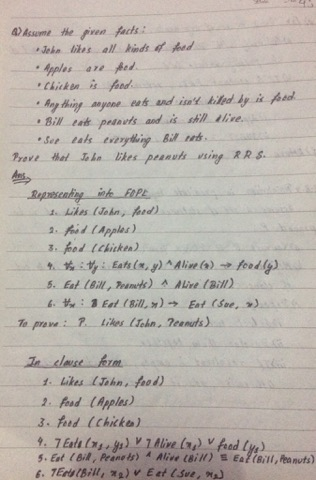Example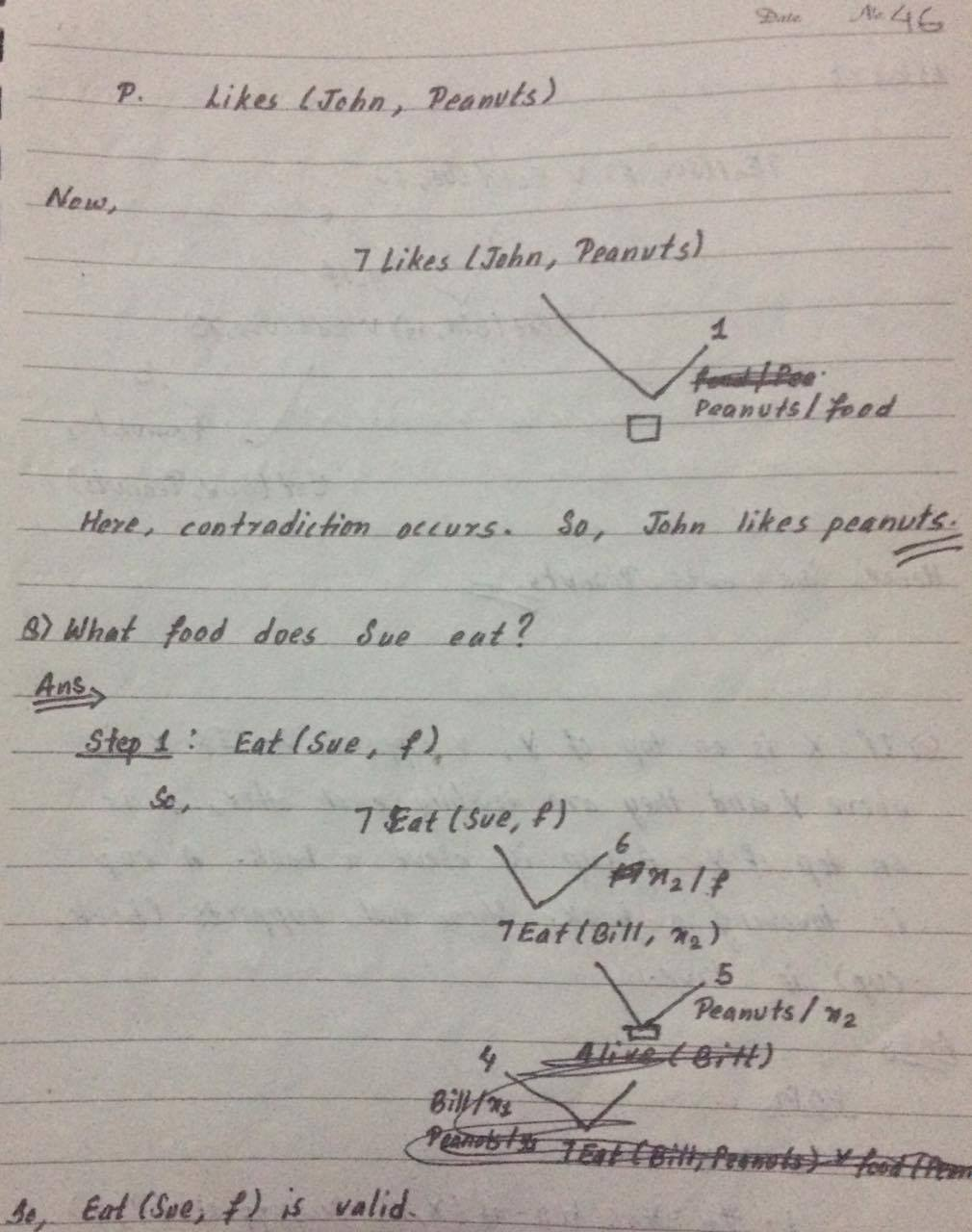Example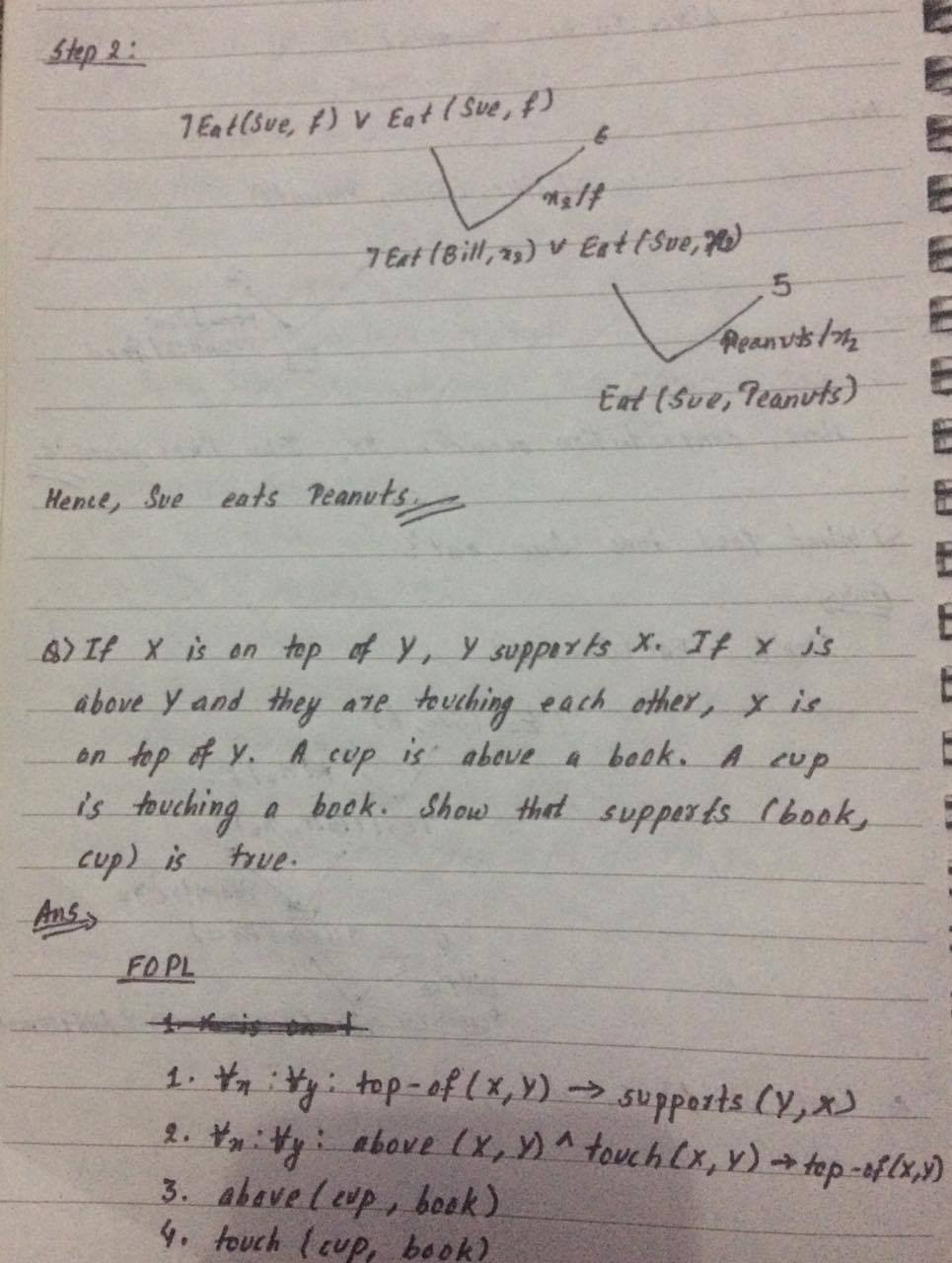Example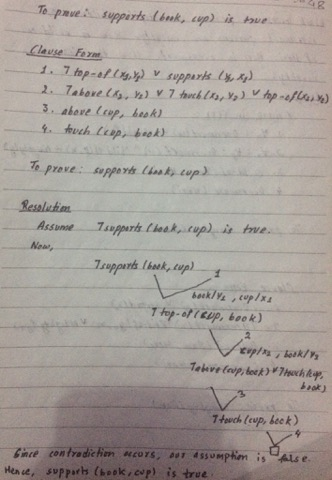Example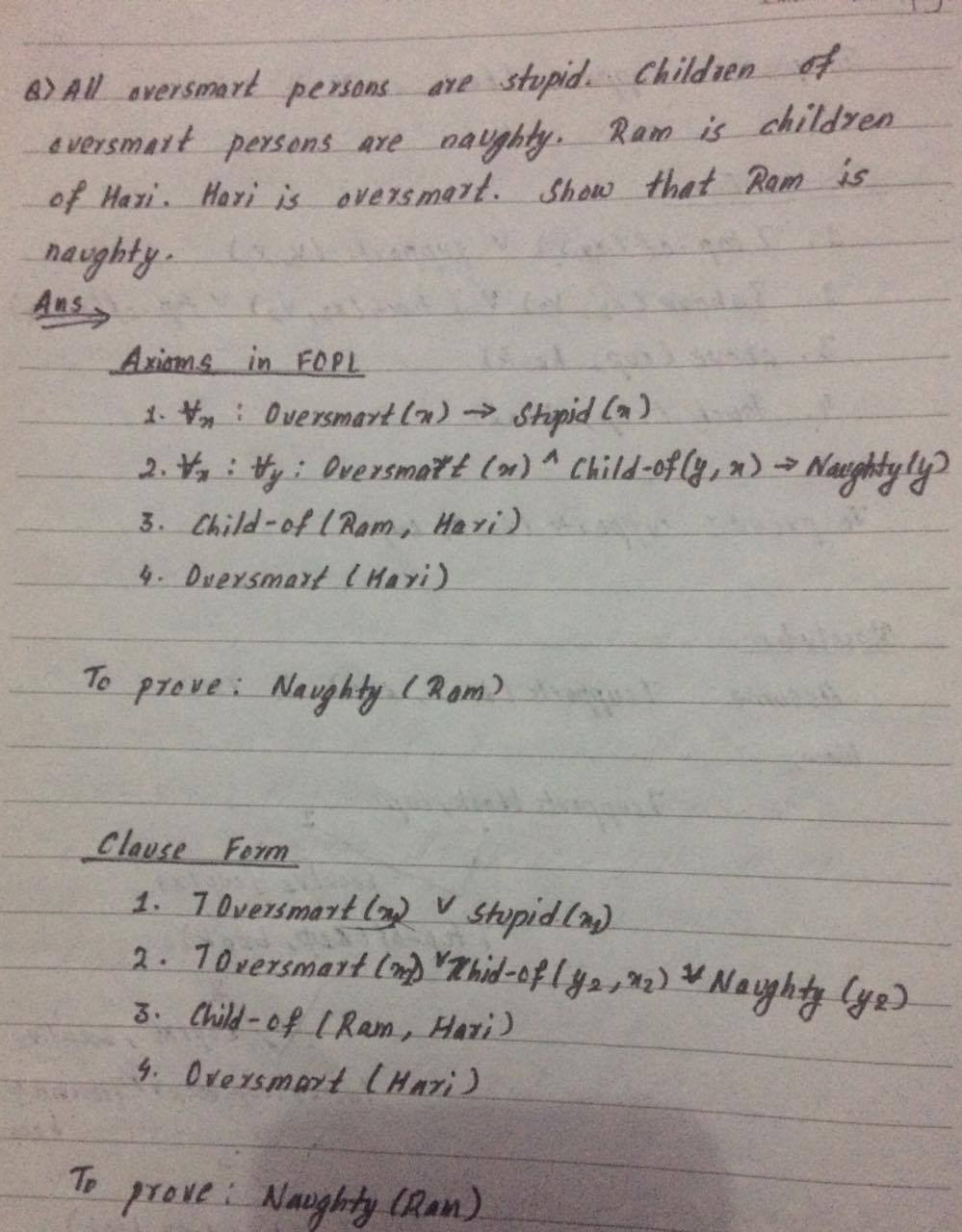Example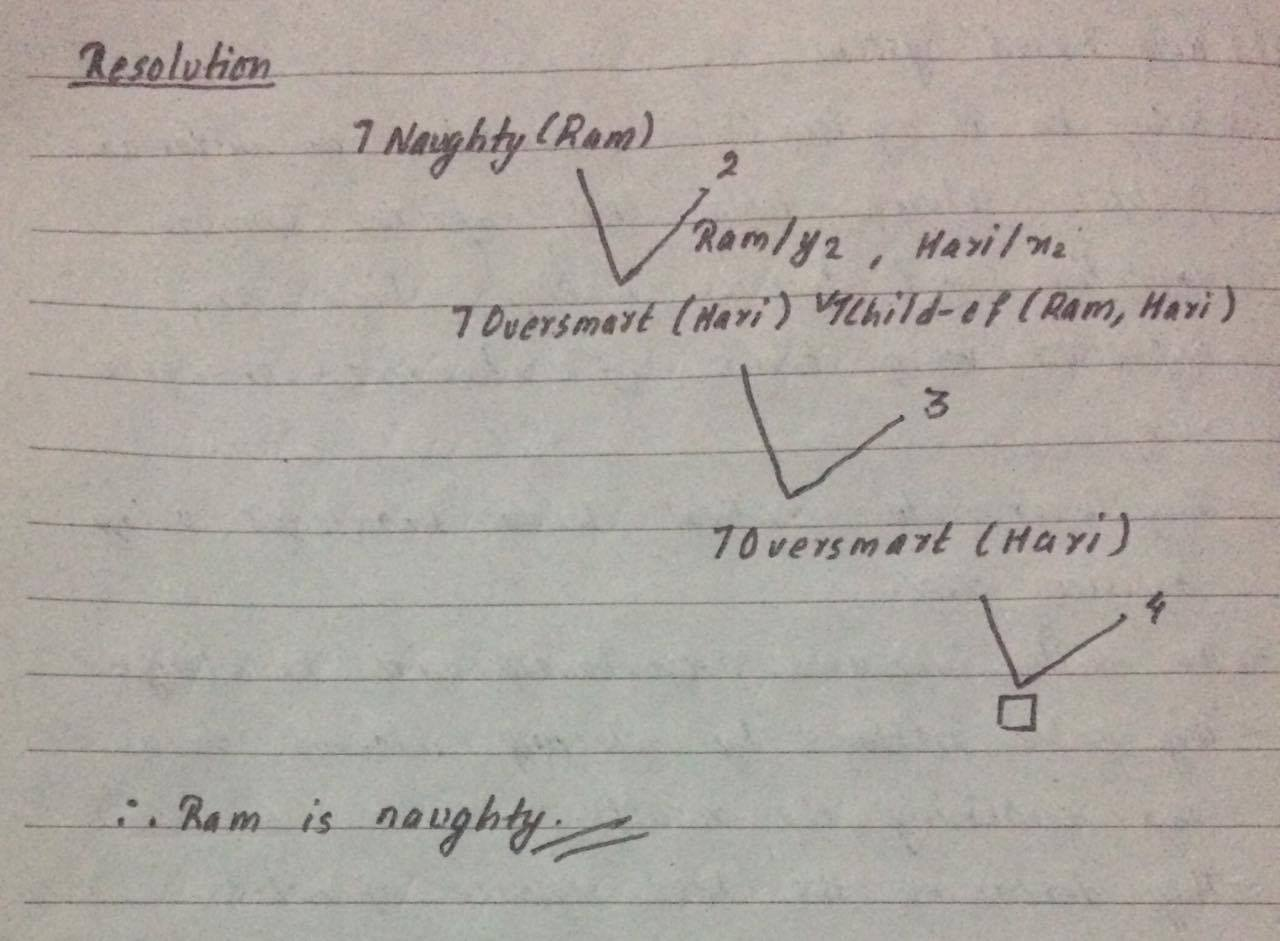Rule Based Deduction System

- Rule based system(RBS) provides an automatic problem solving tools that captures human expertise and decision making which are expressed in terms of antecedent-consequent rules
- They incorporate practical human knowledge using decision rules.
- The skills increases proportionally with knowledge.
- They solve problems by selecting relevant rules and combining the results.
- They determine the best sequence of rules

Forward chaining

- It uses inference rules for reasoning using repeated application of modus poones.
- It starts with available data and uses inference rules until a goal is reached.
- It searches the rules until it finds one in which antecedent is true. It then concludes the consequent and adds new information to its data.
- It is better suited to dynamic situations.
- Used in expert system, production rule system.

Eg:
- The rule base contains the rules as:
P=>Q
L^M=>P
B^L=>M
A^p=>L
A^B=>L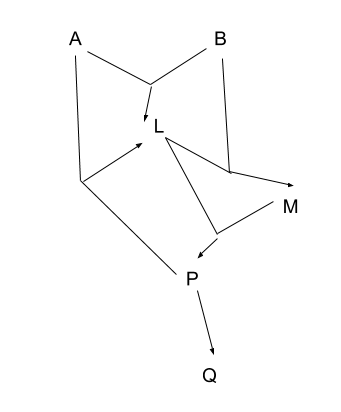Backward chaining

- It starts working backward from the goals. Based on modus ponens rule.
- It employs depth first search strategy
- It starts with goals, searching if data is available which supports any of the consequents.
- It searches until if finds one which has a consequent matching a desired goal.

Q)consider the rule base as:
I) If x cooks and eats flies , then x is a frog.
II) If x chirps and sings, then x is a canary.
III) If x is a frog, then x is green
IV) If x is a canary , then x is yellow.
Conclude color of fritz, given he croaks and eats flies.

Ans:-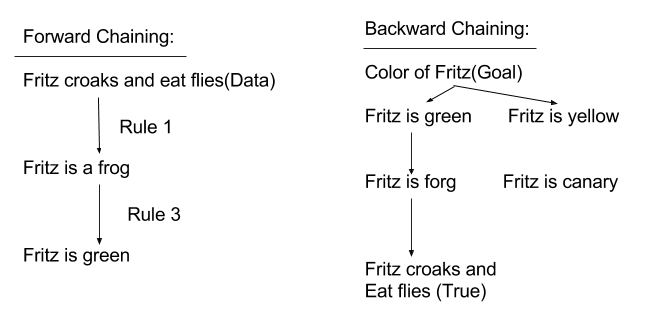Entailment

- It is relationship between current statement and new sentences derived from current sentences.
- It reflects that on facts follow from the others
- Knowledge base (KB) entails sentence if and only if the sentence is true in all worlds where kB is true.
- An inference is called sound if it derives only entailed sentences
- An inference is called complete if it can derive any sentences that are entailed.

Eg:
Consider KB as:
A˅B
˥C˅A
Consider S: A˅B˅C
Show that KB l-S(i.e. KB entails s)

Ans

A B C KB S
F F F F F
F F T F T
F T F T T
F T T F T
T F F T T
T F T T T
T T F T T
T T T T T

Whenever KB is true, s is also true, so, we can say that KB entails S i.e. KB l-S

Statistical Reasoning

- Evidence is collected as the system goes along and to modify the behavior based on the evidence.
- These numerical summery indicate how often an exception of some sort can be expected to occurs.
- It is evidence based reasoning.

Probability and baye’s theorem:-

- Bayesian statistics provides provide statistical theory of evidence, which is based on conditional probability.
- Bayes theorem states that: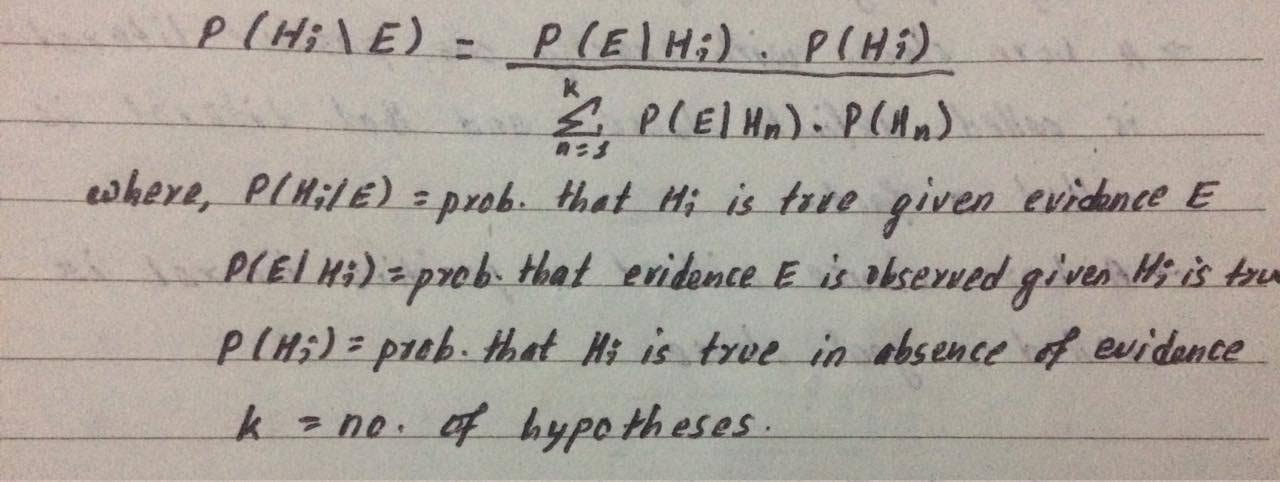Causal network

A casual network or Bayesian network is a probabilistic graphical model that represents a set of random variables and their conditional dependencies via a directed and their conditional dependencies via a directed acyclic graph. The nodes represent the random variables and edges represent conditional dependencies. Each node is associated with a probability function with i/p(a set of values for node’s parent variables) and gives 0/p (probability of variable).

Example::

Suppose two events cause grass to be wet, either sprinkler is on or its raining. Also, suppose when it rains, sprinkler is usually not turned on. This situation can be modeled using Bayesian network or Belief network as follows: